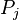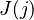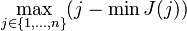# Cognitive load when learning mathematics

• If a proof has steps$P_1, P_2, P_3, \ldots, P_n$ the proof is much easier to follow when the justifications for a step$P_j$ are close to$P_j$. When much earlier stages of the proof are required to understand the current step, the reader has to go back to remember what was done early on. If$J(j)$ is the set of justification numbers used on step$P_j$ (e.g. maybe$J(5) = \{1,3\}$), then the quantity of interest is something like$\max_{j\in \{1,\ldots,n\}}(j - \min J(j))$, which is the most number of steps the proof stretches backwards (or maybe it's the average of this quantity, or the average length of "stretching back"). Kind of related to space requirements for an algorithm and things like peephole optimization.